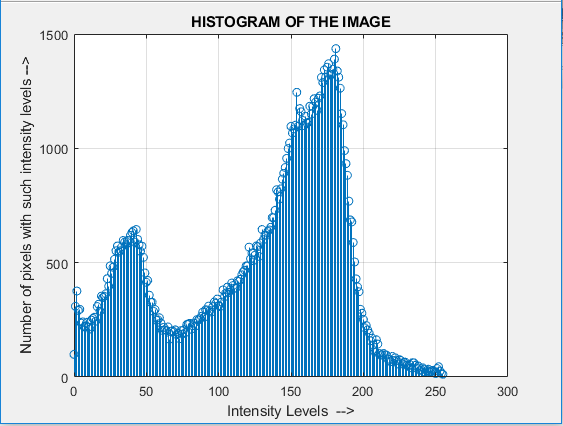# MATLAB | Display histogram of a grayscale Image

An image histogram is chart representation of the distribution of intensities in an Indexed image or grayscale image. It shows how many times each intensity value in image occurs.

Code #1: Display histogram of an image using MATLAB library function.

 `% Read an Image in MATLAB Environment ` `img=imread(``'apple.jpg'``); ` ` `  `% Convert image to grayscale image ` `# ``if` `read image is an RGB image ` `img=rgb2gray(img); ` ` `  `% Show histogram of image ` `# using imhist() ``function` `imhist(img); `

Code #2: Display Histogram of an Image without using MATLAB Library function.

Approach :

• Read the source image file into image matrix
• Convert it to grayscale, if it is an RGB image
• Iterate over image matrix and count the frequency of every possible value of intensity
• plot the counted frequency

 `% Read source image file  ` `img = imread(``'apple.jpg'``); ` ` `  `% Convert image to grayscale image  ` `img=rgb2gray(img); ` ` `  `% get the dimension of the image  ` `[x, y] = size(img); ` ` `  ` `  `% Create a frequency array of size 256 ` `frequency = 1 : 256; ` ` `  `count = 0; ` ` `  `% Iterate over grayscale image matrix  ` `% for every possible intensity value ` `% and count them ` `   `  `for` `i = 1 : 256 ` `    ``for` `j = 1 : x ` `        ``for` `k = 1 : y ` ` `  `            ``% if image pixel value at location (j, k) is i-1 ` `            ``% then increment count ` `            ``if` `img(j, k) == i-1 ` `                    ``count = count + 1; ` `            ``end` `        ``end` `    ``end` `    ``% update ith position of frequency array with count ` `    ``frequency(i) = count; ` ` `  `    ``% reset count ` `    ``count = 0; ` ` `  `end` ` `  ` `  `n = 0 : 255; ` ` `  `% Display Histogram ` `stem(n, frequency); ` ` `  `grid on; ` `ylabel(``'Number of pixels with such intensity levels -->'``); ` `xlabel(``'Intensity Levels  -->'``); ` `title(``'HISTOGRAM OF THE IMAGE'``); `

Input:Output:My Personal Notes arrow_drop_upCheck out this Author's contributed articles.

If you like GeeksforGeeks and would like to contribute, you can also write an article using contribute.geeksforgeeks.org or mail your article to contribute@geeksforgeeks.org. See your article appearing on the GeeksforGeeks main page and help other Geeks.

Please Improve this article if you find anything incorrect by clicking on the "Improve Article" button below.

Article Tags :

1

Please write to us at contribute@geeksforgeeks.org to report any issue with the above content.